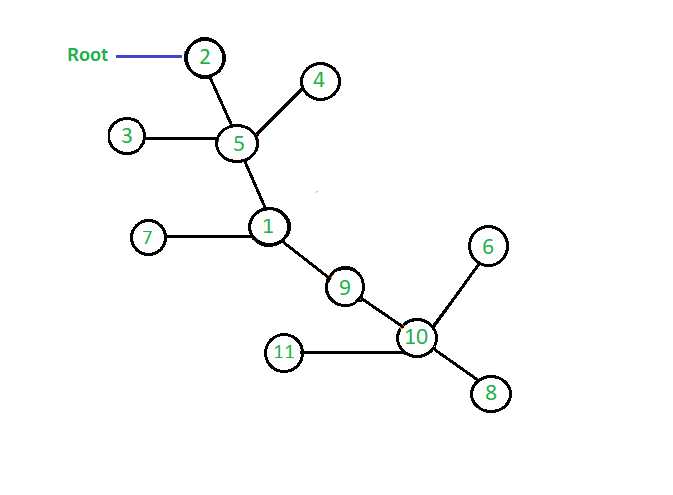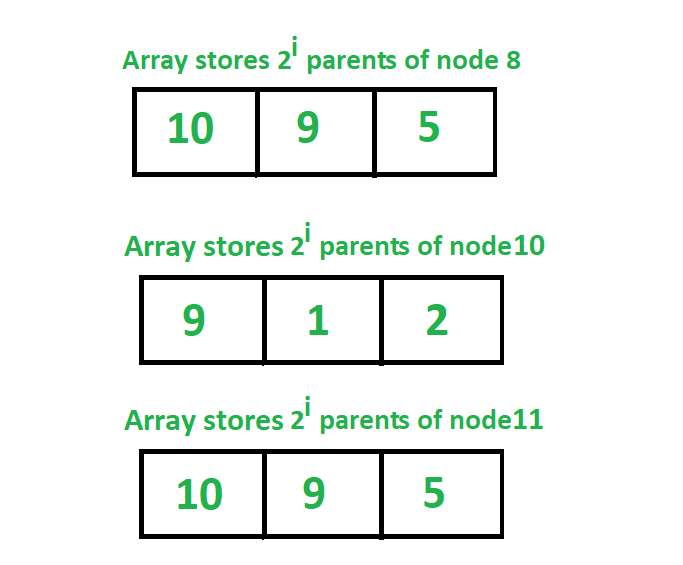# Jump Pointer Algorithm

The Jump pointer algorithm is a design technique for parallel algorithms that operate on pointer structures, such as arrays or linked list. This algorithm is normally used to determine the root of the forest of a rooted tree.

In the jump pointer algorithm, we pre-process a tree so that one can able to answer the queries to find any parent of any node in the tree in time complexity of O(log n).

The jump pointer algorithm associates up to log2n pointers to each vertex of the tree. These pointers are called jump pointers because they jump up the tree towards the root node of the tree. For any node of the processing tree, the algorithm stores an array of length l jumpers where l = log2(depth(v)). The ith element of this array points to the 2ith parent of the node v. This data structure helps us to jump halfway up the tree from any given node. When the algorithm is asked to process a query to find any parent of any node in the tree, we repeatedly jump up the tree using these pointers. The number of jumps will be at most log n and therefore any problem to find the parent of any node in the tree can be answered in O(log n) time complexity.
In jump pointer, there is a pointer from node N to N’s j-th parent, for
j = 1, 2, 4, 8, …, and so on. So we store 2ith parent of each node.

The jump pointer algorithm basically works on the approach of dynamic programming where we use the pre-computed result to find the next result. By doing some simple calculations we can compute the mathematical formula that for any node k, 2jth parent of k is equal to 2j-1th parent of 2j-1th parent of k.
The brief explanation of this formula is given below in the algorithm section.

The most common use of this algorithm is to solve the queries in which we need to find the ancestor of any node in O(log n) time complexity.
Graph to implement Jump Pointer Algorithm:Representation of jump array that stores 2^i th parent of all nodes:Examples:

```
Input: 0th parent of node 2
Output: 0th parent of node 2 is = 2

Input: 2th parent of node 4
Output: 2th parent of node 4 is = 2

Input: 3rd parent of node 8
Output: 3rd parent of node 8 is = 1

```

## Recommended: Please try your approach on {IDE} first, before moving on to the solution.

Algorithm :
Here is the algorithm to implement the jump pointer algorithm to find any parent of any node in the graph. We determine the jump matrix using dynamic programming. Here, we denote the root node as R and initially assume the parent of the root node as 0 which means there is no parent of this node. Now looking at the graph and the array is shown in the above diagram we can easily understand the above formula to determine the 2ith parent of each node. If we look at the node with value 8 we can see that its 20th parent is 10, now to find its 21th parent we see that it’s 21th parent is 20th parent of the node with value 10 and here 10 is 20th parent of 8 it means 21th parent of node 8 is 20th parent of 20th parent of node 8. Similarly we can also see that 22th parent of node 8 is 5 which is 21th parent of 21th parent of node 8 i.e 21th parent of node with value 9.
Thus in this way we can compute the array of jump pointers for all the nodes to store their 2ith parents.
Below is the pseudo code to calculate the jump pointer matrix that store 2ith parent of all the nodes in the tree.

```jump[k][j] =   it points 2^jth parent of k
=  2^j-1th parent of (2^j-1th parent of k)
=  jump[jump[i][j-1][j-1]

```

Implementation: Below is the code to implement the above algorithm to find any parent of any node in O( logn ) time complexity.

 `// C++ program to implement Jump pointer algorithm ` `#include ` `using` `namespace` `std; ` ` `  `int` `R = 0; ` `// n -> it represent total number of nodes ` `// len -> it is the maximum length of array ` `// to hold parent of each node. ` `// In worst case, the highest value of ` `// parent a node can have is n-1. ` `// 2 ^ len <= n-1 ` `// len = O(log2n) ` `int` `getLen(``int` `n) ` `{ ` `    ``int` `len = (``int``)(``log``(n) / ``log``(2)) + 1; ` `    ``return` `len; ` `} ` ` `  `// jump represent 2D matrix to hold parent of node in jump matrix ` `// here we pass reference of 2D matrix so that the change made ` `// occur directly to the original matrix ` `// len is same as defined above ` `// n is total nodes in graph ` `void` `set_jump_pointer(vector >& jump, ` `                      ``int``* node, ``int` `len, ``int` `n) ` `{ ` `    ``for` `(``int` `j = 1; j <= len; j++) ` `        ``for` `(``int` `i = 0; i < n; i++) ` `            ``jump[node[i]][j] = jump[jump[node[i]][j - 1]][j - 1]; ` `} ` ` `  `// c -> it represent child ` `// p -> it represent parent ` `// i -> it represent node number ` `// p=0 means the node is root node ` `// here also we pass reference of 2D matrix ` `// and depth vector so that the change made ` `// occur directly to the original matrix and original vector ` `void` `constructGraph(vector >& jump, ` `                    ``int``* node, ``int``* isNode, ``int` `c, ``int` `p, ``int` `i) ` `{ ` `    ``// enter the node in node array ` `    ``// it stores all the nodes in the graph ` `    ``node[i] = c; ` ` `  `    ``// to confirm that no child node have 2 parents ` `    ``if` `(isNode == 0) { ` `        ``isNode = 1; ` `        ``// make parent of x as y ` `        ``jump = p; ` `    ``} ` `    ``return``; ` `} ` ` `  `// function to jump to Lth parent of any node ` `void` `jumpPointer(vector >& jump, ` `                 ``int``* isNode, ``int` `x, ``int` `L) ` `{ ` `    ``int` `j = 0, n = x, k = L; ` ` `  `    ``// to check if node is present in graph or not ` `    ``if` `(isNode[x] == 0) { ` `        ``cout << ``"Node is not present in graph "` `<< endl; ` `        ``return``; ` `    ``} ` ` `  `    ``// in this loop we decrease the value of L by L/2 and ` `    ``// increment j by 1 after each iteration, and check for set bit ` `    ``// if we get set bit then we update x with jth parent of x ` `    ``// as L becomes less than or equal to zero means ` `    ``// we have jumped to Lth parent of node x ` `    ``while` `(L > 0) { ` `        ``// to check if last bit is 1 or not ` `        ``if` `(L & 1) ` `            ``x = jump[x][j]; ` ` `  `        ``// use of shift operator to make ` `        ``// L = L/2 after every iteration ` `        ``L = L >> 1; ` `        ``j++; ` `    ``} ` ` `  `    ``cout << k << ``"th parent of node "` `<< n  ` `                   ``<< ``" is = "` `<< x << endl; ` ` `  `    ``return``; ` `} ` ` `  `// Driver code ` `int` `main() ` `{ ` `    ``// n represent number of nodes ` `    ``int` `n = 11; ` ` `  `    ``// initialization of parent matrix ` `    ``// suppose max range of a node is up to 1000 ` `    ``// if there are 1000 nodes than also ` `    ``// length of jump matrix will not exceed 10 ` `    ``vector > jump(1000, vector<``int``>(10)); ` ` `  `    ``// node array is used to store all nodes ` `    ``int``* node = ``new` `int``; ` ` `  `    ``// isNode is an array to check whether ` `    ``// a node is present in graph or not ` `    ``int``* isNode = ``new` `int``; ` ` `  `    ``// memset function to initialize isNode array with 0 ` `    ``memset``(isNode, 0, 1000 * ``sizeof``(``int``)); ` ` `  `    ``// function to calculate len ` `    ``// len -> it is the maximum length of ` `    ``// array to hold parent of each node. ` `    ``int` `len = getLen(n); ` ` `  `    ``// R stores root node ` `    ``R = 2; ` ` `  `    ``// construction of graph ` `    ``// here 0 represent that the node is root node ` `    ``constructGraph(jump, node, isNode, 2, 0, 0); ` `    ``constructGraph(jump, node, isNode, 5, 2, 1); ` `    ``constructGraph(jump, node, isNode, 3, 5, 2); ` `    ``constructGraph(jump, node, isNode, 4, 5, 3); ` `    ``constructGraph(jump, node, isNode, 1, 5, 4); ` `    ``constructGraph(jump, node, isNode, 7, 1, 5); ` `    ``constructGraph(jump, node, isNode, 9, 1, 6); ` `    ``constructGraph(jump, node, isNode, 10, 9, 7); ` `    ``constructGraph(jump, node, isNode, 11, 10, 8); ` `    ``constructGraph(jump, node, isNode, 6, 10, 9); ` `    ``constructGraph(jump, node, isNode, 8, 10, 10); ` ` `  `    ``// function to pre compute jump matrix ` `    ``set_jump_pointer(jump, node, len, n); ` ` `  `    ``// query to jump to parent using jump pointers ` `    ``// query to jump to 1st parent of node 2 ` `    ``jumpPointer(jump, isNode, 2, 0); ` ` `  `    ``// query to jump to 2nd parent of node 4 ` `    ``jumpPointer(jump, isNode, 4, 2); ` ` `  `    ``// query to jump to 3rd parent of node 8 ` `    ``jumpPointer(jump, isNode, 8, 3); ` ` `  `    ``// query to jump to 5th parent of node 20 ` `    ``jumpPointer(jump, isNode, 20, 5); ` ` `  `    ``return` `0; ` `} `

Output:

```0th parent of node 2 is = 2
2th parent of node 4 is = 2
3th parent of node 8 is = 1
Node is not present in graph
```

Attention reader! Don’t stop learning now. Get hold of all the important DSA concepts with the DSA Self Paced Course at a student-friendly price and become industry ready.

My Personal Notes arrow_drop_upCheck out this Author's contributed articles.

If you like GeeksforGeeks and would like to contribute, you can also write an article using contribute.geeksforgeeks.org or mail your article to contribute@geeksforgeeks.org. See your article appearing on the GeeksforGeeks main page and help other Geeks.

Please Improve this article if you find anything incorrect by clicking on the "Improve Article" button below.

Improved By : ManasChhabra2

1

Please write to us at contribute@geeksforgeeks.org to report any issue with the above content.